Read an Intro to Finding Area | for kids 3rd, 4th, & 5th Grade
1%
It was processed successfully!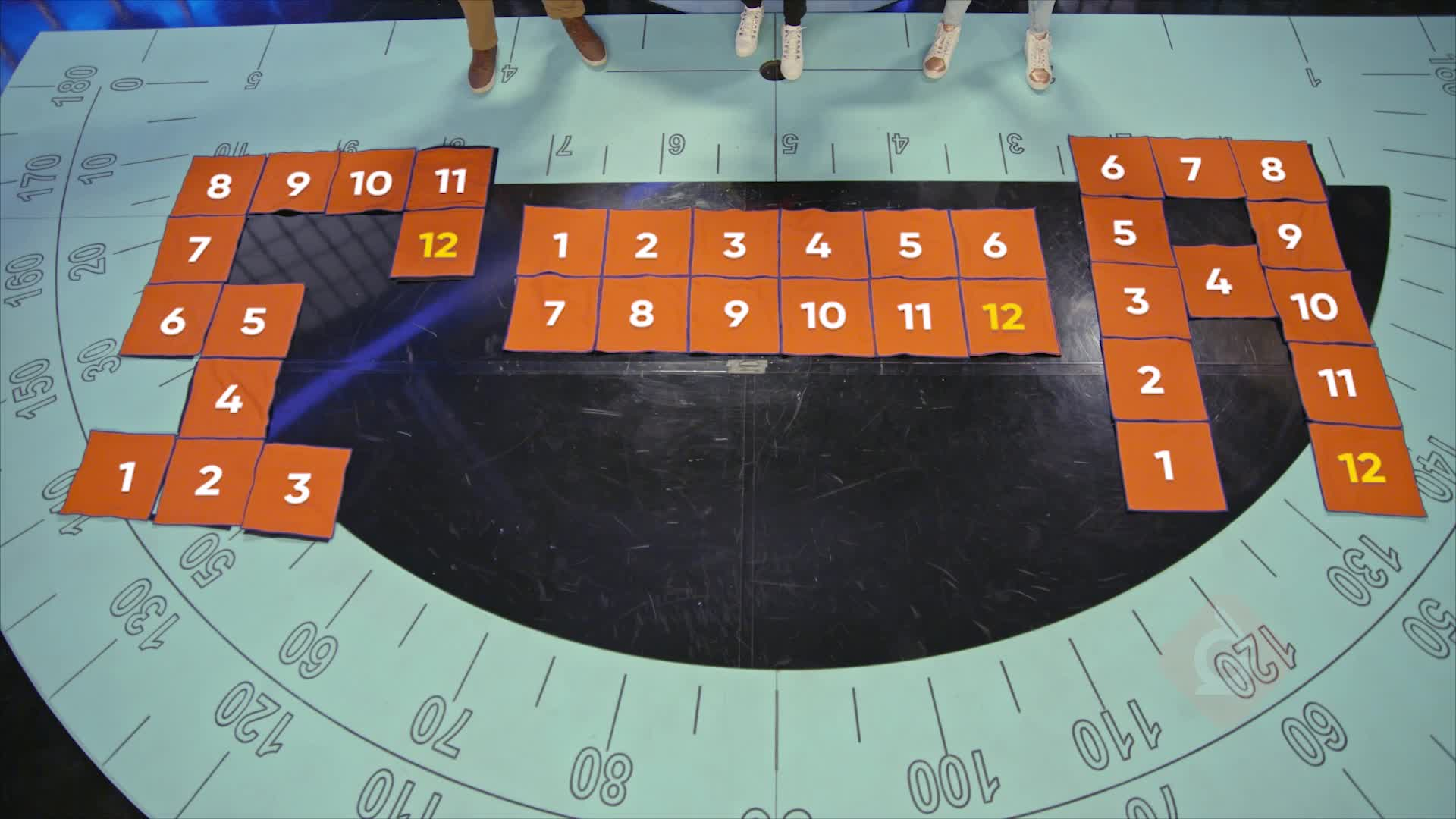WHAT IS INTRO TO FINDING AREA?

You will learn what area is, how area is measured, how to find area by counting square units, and how to find the area of rectangles using addition or multiplication. You will also learn that area is additive and shapes can be composed and decomposed to find the area of shapes formed by rectangles.

To better understand finding area…

WHAT IS INTRO TO FINDING AREA?. You will learn what area is, how area is measured, how to find area by counting square units, and how to find the area of rectangles using addition or multiplication. You will also learn that area is additive and shapes can be composed and decomposed to find the area of shapes formed by rectangles.  To better understand finding area…

## LET’S BREAK IT DOWN!

### Somersaults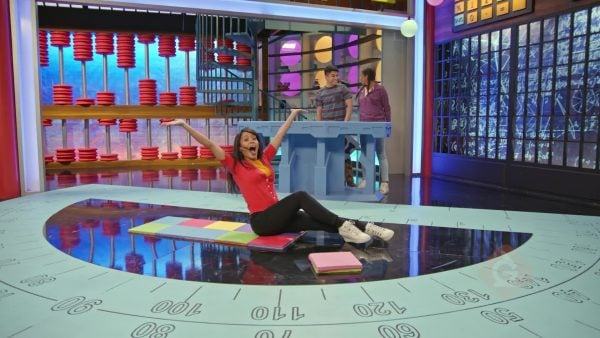Area is measured in square units. Let’s say you want to make a floor mat for a tumbling class. You put 5 rows that each have 2 square pads. You can count the number of squares to find the area you can tumble on and find that the area is 10 square units. Try this one yourself: You lay 24 square pads in a rectangular mat for a wrestling class. Then, you pick up 4 of the pads. What is the area of the new mat?

Somersaults Area is measured in square units. Let’s say you want to make a floor mat for a tumbling class. You put 5 rows that each have 2 square pads. You can count the number of squares to find the area you can tumble on and find that the area is 10 square units. Try this one yourself: You lay 24 square pads in a rectangular mat for a wrestling class. Then, you pick up 4 of the pads. What is the area of the new mat?

### Defenders of the Area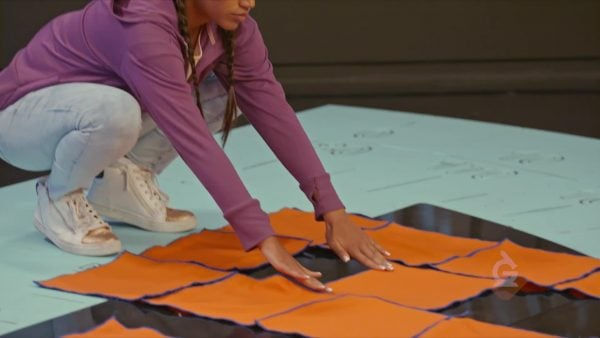Different shapes can have the same area, as long as the same number of square units are needed to fill the space. You can also add or remove square units for a larger or smaller area. Let’s say you have a cape that is made of 27 squares of fabric of the same size. It is 9 squares long and 3 squares wide. You remove 6 squares from the bottom of the cape and attach them to a side to use as a hood. The area of your cape is still 27 square units. Try this one yourself: You use 12 square units of fabric to make a cape that is 3 squares long and 4 squares wide. Your cape is not long enough, so you add 9 more squares at the bottom. What is the area of your cape now?

Defenders of the Area Different shapes can have the same area, as long as the same number of square units are needed to fill the space. You can also add or remove square units for a larger or smaller area. Let’s say you have a cape that is made of 27 squares of fabric of the same size. It is 9 squares long and 3 squares wide. You remove 6 squares from the bottom of the cape and attach them to a side to use as a hood. The area of your cape is still 27 square units. Try this one yourself: You use 12 square units of fabric to make a cape that is 3 squares long and 4 squares wide. Your cape is not long enough, so you add 9 more squares at the bottom. What is the area of your cape now?

### Poster Array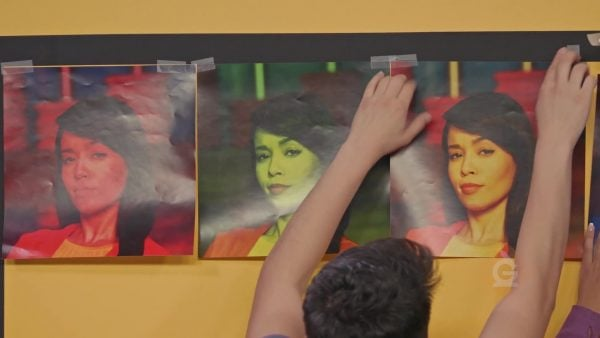The area of a rectangle or square can be thought of as an array, with rows and columns of square units. To find the number of square units in the shape, you can multiply the number of columns by the number of rows. This is the same as multiplying the length by the width. Let’s say you have square posters that you are using to cover a wall. The wall fits 5 posters high and 5 posters wide. You can multiply 5 times 5 to find that the total area of the wall is 25 square posters. Try this one yourself: One-foot square posters are used to cover a wall. The wall is 6 posters high and 4 posters wide. What is the area covered by the posters?

Poster Array The area of a rectangle or square can be thought of as an array, with rows and columns of square units. To find the number of square units in the shape, you can multiply the number of columns by the number of rows. This is the same as multiplying the length by the width. Let’s say you have square posters that you are using to cover a wall. The wall fits 5 posters high and 5 posters wide. You can multiply 5 times 5 to find that the total area of the wall is 25 square posters. Try this one yourself: One-foot square posters are used to cover a wall. The wall is 6 posters high and 4 posters wide. What is the area covered by the posters?

### Pixel Art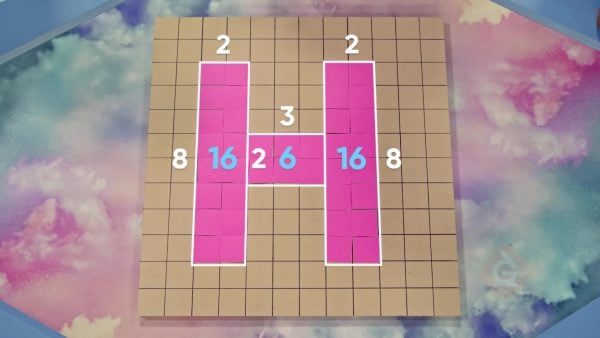Shapes are not always perfect squares or rectangles. Sometimes you can break shapes apart into squares and rectangles to find their area. Let’s say you have a T-shaped figure that can be broken into a 2 × 2 square and a 6 × 2 rectangle. You can multiply to find that the area of the square is 4 square units and the area of the rectangle is 12 square units. Then, you can add the two areas to find that the area of the full shape is 4 + 12 = 16 square units. Try this one yourself: You have a U-shaped figure that can be broken into 2 rectangles that are 6 units long and 3 units wide and one square with a side length of 3 units. What is the area of the figure?

Pixel Art Shapes are not always perfect squares or rectangles. Sometimes you can break shapes apart into squares and rectangles to find their area. Let’s say you have a T-shaped figure that can be broken into a 2 × 2 square and a 6 × 2 rectangle. You can multiply to find that the area of the square is 4 square units and the area of the rectangle is 12 square units. Then, you can add the two areas to find that the area of the full shape is 4 + 12 = 16 square units. Try this one yourself: You have a U-shaped figure that can be broken into 2 rectangles that are 6 units long and 3 units wide and one square with a side length of 3 units. What is the area of the figure?

## INTRO TO FINDING AREA VOCABULARY

Area
Area is the amount of space inside a closed, two-dimensional shape.
Square
A square is a polygon with four sides that are the same length and four right angles.
Rectangle
A rectangle is a polygon with four sides and four right angles.
Unit of measurement
A way to communicate the size of a measurement.
Square unit
A square unit is a square with side lengths of 1 unit that is used to measure the area of a shape.
Formula
A rule or fact written with mathematical symbols. The formula for the area of a rectangle is length times width.
Made by arranging items or shapes into equal rows and columns.
A shape that can be made using smaller shapes. For example, an L can be made using two rectangles. The area of a composite shape is the sum of the areas of the smaller shapes.

## INTRO TO FINDING AREA DISCUSSION QUESTIONS

### What is area? How is it measured?

Area is the amount of space inside a shape. Area is measured using square units.

### How could you find area using grid paper?

You could draw a shape on the paper and count the number of square units inside the shape.

### How can an array help you find the area of a rectangle that is 6 inches long and 8 inches wide?

You can find the number of cells in an array by multiplying the number of rows by the number of columns. This is the same idea as finding area, but the cells are square inches. 6 inches times 8 inches is 48 square inches.

### How can you find the area of a shape that is not a square or rectangle, such as an L-shape or T-shape?

You can break the shape up into smaller pieces that are squares and rectangles, find the area of the smaller pieces, and then add them together. An L or a T can each be broken into two pieces that are rectangles.

### Garrett puts square photos in a shape that is 8 photos wide and 10 photos tall. Lars takes the same photos and puts them in a shape that is 12 photos wide and 5 photos tall. How many of the photos does Lars have left over? Explain how you know.

Lars has 20 photos left over. Garrett uses 8 × 10 = 80 photos. Lars only uses 12 × 5 = 60 photos. 80 – 60 = 20 photos left over.
X

## Success

We’ve sent you an email with instructions how to reset your password.
Ok

## Choose Your Free Trial Period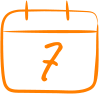### 7 DaysContinue to Lessons### 90 DaysGet 90 days free by inviting other teachers to try it too.

Share with Teachers

## Get 90 Days Free

### By inviting 4 other teachers to try it too.

4 required

Skip, I will use a 7 days free trial

## Thank You!

Enjoy your free 90 days trial